# Drop rows in PySpark DataFrame with condition

• Last Updated : 18 Jul, 2021

In this article, we are going to drop the rows in PySpark dataframe. We will be considering most common conditions like dropping rows with Null values, dropping duplicate rows, etc. All these conditions use different functions and we will discuss these in detail.

We will cover the following topics:

• Drop rows with condition using where() and filter() keyword.
• Drop rows with NA or missing values
• Drop rows with Null values
• Drop duplicate rows.
• Drop duplicate rows based on column

## Python3

 `# importing module``import` `pyspark`` ` `# importing sparksession from pyspark.sql module``from` `pyspark.sql ``import` `SparkSession`` ` `# creating sparksession and giving an app name``spark ``=` `SparkSession.builder.appName(``'sparkdf'``).getOrCreate()`` ` `# list  of students  data``data ``=` `[[``"1"``, ``"sravan"``, ``"vignan"``],``        ``[``"2"``, ``"ojaswi"``, ``"vvit"``],``        ``[``"3"``, ``"rohith"``, ``"vvit"``],``        ``[``"4"``, ``"sridevi"``, ``"vignan"``],``        ``[``"6"``, ``"ravi"``, ``"vrs"``],``        ``[``"5"``, ``"gnanesh"``, ``"iit"``]]`` ` `# specify column names``columns ``=` `[``'ID'``, ``'NAME'``, ``'college'``]`` ` `# creating a dataframe from the lists of data``dataframe ``=` `spark.createDataFrame(data, columns)`` ` `print``(``'Actual data in dataframe'``)``dataframe.show()`

Output: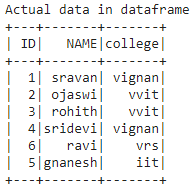### Drop rows with condition using where() and filter() Function

Here we are going to drop row with the condition using where() and filter() function.

where(): This function is used to check the condition and give the results. That means it drops the rows based on the condition

Syntax: dataframe.where(condition)

filter(): This function is used to check the condition and give the results, Which means it drops the rows based on the condition.

Syntax: dataframe.filter(condition)

Example 1: Using Where()

Python program to drop rows where ID less than 4

## Python3

 `# drop rows with id less than 4``dataframe.where(dataframe.``ID``>``4``).show()`

Output: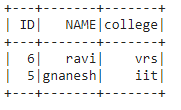Drop rows with college ‘vrs’:

## Python3

 `# drop rows with college vrs``dataframe.where(dataframe.college !``=` `'vrs'``).show()`

Output: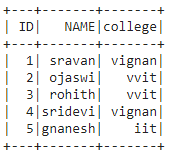Example 2: Using filter() function

Python program to drop rows with id=4

## Python3

 `# drop rows with id 4``dataframe.``filter``(dataframe.``ID``!``=``'4'``).show()`

Output: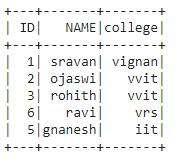### Drop rows with NA values using dropna

NA values are the missing value in the dataframe, we are going to drop the rows having the missing values. They are represented as null, by using dropna() method we can filter the rows.

Syntax: dataframe.dropna()

## Python3

 `# importing module``import` `pyspark`` ` `# importing sparksession from pyspark.sql module``from` `pyspark.sql ``import` `SparkSession`` ` `# creating sparksession and giving an app name``spark ``=` `SparkSession.builder.appName(``'sparkdf'``).getOrCreate()`` ` `# list  of employee data with 5 row values``data ``=` `[[``"1"``, ``"sravan"``, ``"company 1"``],``        ``[``"2"``, ``"ojaswi"``, ``"company 2"``],``        ``[``None``, ``"bobby"``, ``"company 3"``],``        ``[``"1"``, ``"sravan"``, ``"company 1"``],``        ``[``"2"``, ``"ojaswi"``, ``None``],``        ``[``"4"``, ``"rohith"``, ``"company 2"``],``        ``[``"5"``, ``"gnanesh"``, ``"company 1"``],``        ``[``"2"``, ``None``, ``"company 2"``],``        ``[``"3"``, ``"bobby"``, ``"company 3"``],``        ``[``"4"``, ``"rohith"``, ``"company 2"``]]`` ` `# specify column names``columns ``=` `[``'Employee ID'``, ``'Employee NAME'``, ``'Company Name'``]`` ` `# creating a dataframe from the lists of data``dataframe ``=` `spark.createDataFrame(data, columns)`` ` `# display actual dataframe``dataframe.show()`` ` `# drop missing values``dataframe ``=` `dataframe.dropna()`` ` `# display  dataframe after dropping null values``dataframe.show()`

Output: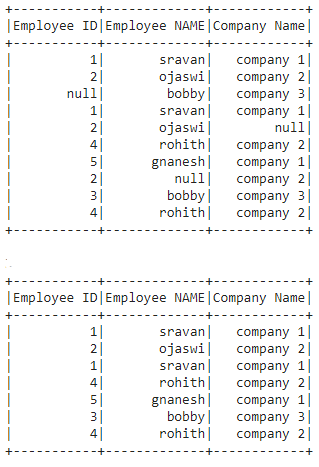### Drop rows with Null values using isNotNull

Here we are dropping the rows with null values, we are using isNotNull() function to drop the rows

Syntax: dataframe.where(dataframe.column.isNotNull())

Python program to drop null values based on a particular column

## Python3

 `# importing module``import` `pyspark`` ` `# importing sparksession from pyspark.sql module``from` `pyspark.sql ``import` `SparkSession`` ` `# creating sparksession and giving an app name``spark ``=` `SparkSession.builder.appName(``'sparkdf'``).getOrCreate()`` ` `# list  of employee data with 5 row values``data ``=` `[[``"1"``, ``"sravan"``, ``"company 1"``],``        ``[``"2"``, ``"ojaswi"``, ``"company 2"``],``        ``[``None``, ``"bobby"``, ``"company 3"``],``        ``[``"1"``, ``"sravan"``, ``"company 1"``],``        ``[``"2"``, ``"ojaswi"``, ``None``],``        ``[``None``, ``"rohith"``, ``"company 2"``],``        ``[``"5"``, ``"gnanesh"``, ``"company 1"``],``        ``[``"2"``, ``None``, ``"company 2"``],``        ``[``"3"``, ``"bobby"``, ``"company 3"``],``        ``[``"4"``, ``"rohith"``, ``"company 2"``]]`` ` `# specify column names``columns ``=` `[``'ID'``, ``'Employee NAME'``, ``'Company Name'``]`` ` `# creating a dataframe from the lists of data``dataframe ``=` `spark.createDataFrame(data, columns)``dataframe.show()`` ` `# removing null values in ID column``dataframe.where(dataframe.``ID``.isNotNull()).show()`

Output: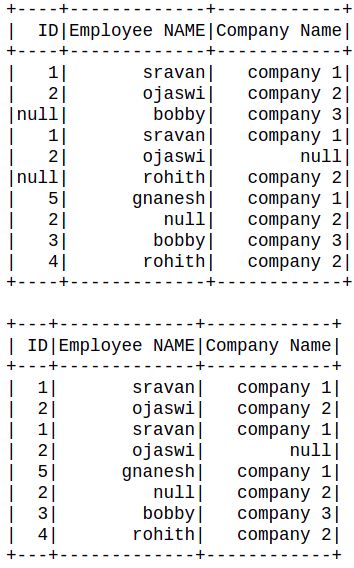### Drop duplicate rows

Duplicate rows mean rows are the same among the dataframe, we are going to remove those rows by using dropDuplicates() function.

Example 1: Python code to drop duplicate rows.

Syntax: dataframe.dropDuplicates()

## Python3

 `# importing module``import` `pyspark`` ` `# importing sparksession from pyspark.sql module``from` `pyspark.sql ``import` `SparkSession`` ` `# creating sparksession and giving an app name``spark ``=` `SparkSession.builder.appName(``'sparkdf'``).getOrCreate()`` ` `# list  of employee data with 5 row values``data ``=` `[[``"1"``, ``"sravan"``, ``"company 1"``],``        ``[``"2"``, ``"ojaswi"``, ``"company 2"``],``        ``[``"3"``, ``"bobby"``, ``"company 3"``],``        ``[``"1"``, ``"sravan"``, ``"company 1"``],``        ``[``"2"``, ``"ojaswi"``, ``"company 2"``],``        ``[``"6"``, ``"rohith"``, ``"company 2"``],``        ``[``"5"``, ``"gnanesh"``, ``"company 1"``],``        ``[``"2"``, ``"ojaswi"``, ``"company 2"``],``        ``[``"3"``, ``"bobby"``, ``"company 3"``],``        ``[``"4"``, ``"rohith"``, ``"company 2"``]]`` ` `# specify column names``columns ``=` `[``'ID'``, ``'Employee NAME'``, ``'Company Name'``]`` ` `# creating a dataframe from the lists of data``dataframe ``=` `spark.createDataFrame(data, columns)``dataframe.show()`` ` `# remove the duplicates``dataframe.dropDuplicates().show()`

Output: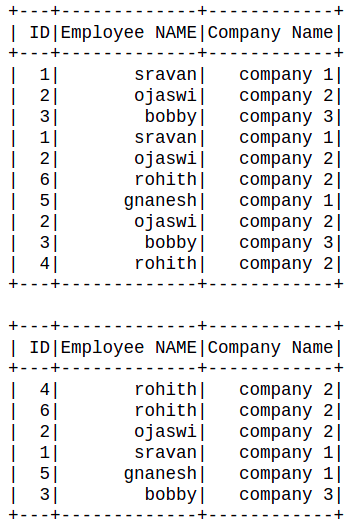Example 2: Drop duplicates based on the column name.

Syntax: dataframe.dropDuplicates([‘column_name’])

Python code to drop duplicates based on employee name

## Python3

 `# remove the duplicates``dataframe.dropDuplicates([``'Employee NAME'``]).show()`

Output: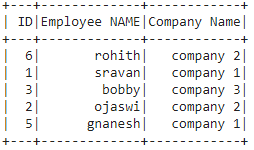### Remove duplicate rows by using a distinct function

We can remove duplicate rows by using a distinct function.

Syntax: dataframe.distinct()

## Python3

 `# importing module``import` `pyspark`` ` `# importing sparksession from pyspark.sql module``from` `pyspark.sql ``import` `SparkSession`` ` `# creating sparksession and giving an app name``spark ``=` `SparkSession.builder.appName(``'sparkdf'``).getOrCreate()`` ` `# list  of employee data with 5 row values``data ``=` `[[``"1"``, ``"sravan"``, ``"company 1"``],``        ``[``"2"``, ``"ojaswi"``, ``"company 2"``],``        ``[``"3"``, ``"bobby"``, ``"company 3"``],``        ``[``"1"``, ``"sravan"``, ``"company 1"``],``        ``[``"2"``, ``"ojaswi"``, ``"company 2"``],``        ``[``"6"``, ``"rohith"``, ``"company 2"``],``        ``[``"5"``, ``"gnanesh"``, ``"company 1"``],``        ``[``"2"``, ``"ojaswi"``, ``"company 2"``],``        ``[``"3"``, ``"bobby"``, ``"company 3"``],``        ``[``"4"``, ``"rohith"``, ``"company 2"``]]`` ` `# specify column names``columns ``=` `[``'ID'``, ``'Employee NAME'``, ``'Company Name'``]`` ` `# creating a dataframe from the lists of data``dataframe ``=` `spark.createDataFrame(data, columns)`` ` `# remove the duplicates by using distinct function``dataframe.distinct().show()`

Output: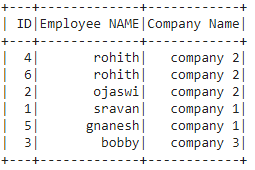My Personal Notes arrow_drop_up